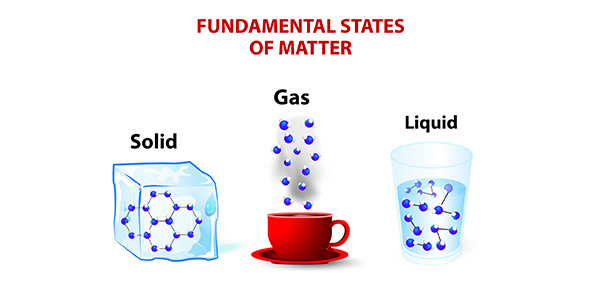# Matter And Solutions Quiz

15 QuestionsSettings.

Related Topics
• 1.
A substance that is made up of only one kind of atom is a(an)
• A.

Compound

• B.

Homogenous Mixture

• C.

Element

• D.

Solution

• 2.
A mixture that appears to contain only one substance is a(an)
• A.

Homogenous Mixture

• B.

Heterogenous Mixture

• C.

Compound

• D.

Element

• 3.
Which of the following is a chemical change?
• A.

Ice melting

• B.

Ice Carved

• C.

Water boiling

• D.

Water breaking down into Hydrogen and Oxygen

• 4.
Which state of matter will hold its shape without a container?
• A.

Solid

• B.

Liquid

• C.

Gas

• D.

Plasma

• 5.
A liquid changes rapidly into a gas at the liquid’s
• A.

Boiling Point

• B.

Freezing Point

• C.

Melting Point

• D.

Condensation Point

• 6.
Grinding quartz crystals down to produce sand is an example of a
• A.

Change of state

• B.

Chemical Change

• C.

Chemical reaction

• D.

Physical Change

• 7.
Which of the following is not a potential sign of chemical change?
• A.

Release of a gas

• B.

Evaporation of Water

• C.

Change of color

• D.

Production of gas

• 8.
A gas has....
• A.

A definite volume but no definite shape.

• B.

A definite shape but no definite volume

• C.

No definite shape or definite volume

• D.

A definite volume and definite shape

• 9.
Matter that has a definite volume but no definite shape is a
• A.

Liquid

• B.

Solid

• C.

Gas

• D.

Plasma

• 10.
The phase change in which a substance changes from a solid to a liquid is
• A.

Freezing

• B.

Melting

• C.

Sublimation

• D.

Suspension

• 11.
Fog is an example of a ____.
• A.

Colloid

• B.

Compound

• C.

Solution

• D.

Substance

• 12.
____ is another name for a homogeneous mixture.
• A.

Liquid

• B.

Solution

• C.

Substance

• D.

Suspension

• 13.
The scattering of light by colloids is called ____.
• A.

Air Pollution

• B.

Conservation

• C.

Suspension

• D.

The Tyndall Effect

• 14.
Matter that has a definite volume and a definite shape is a ____.
• A.

Gas

• B.

Liquid

• C.

Plasma

• D.

Solid

• 15.
The phase change in which a substance changes from a liquid to a gas is
• A.

Deposition

• B.

Sublimation

• C.

Condensation

• D.

Vaporization